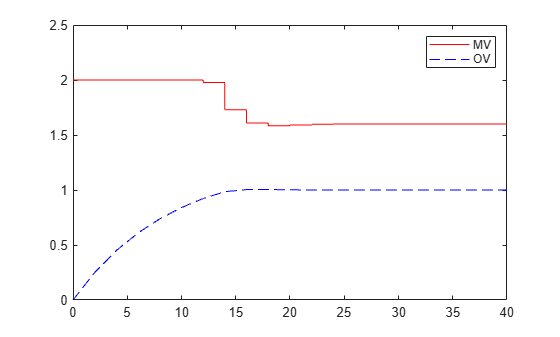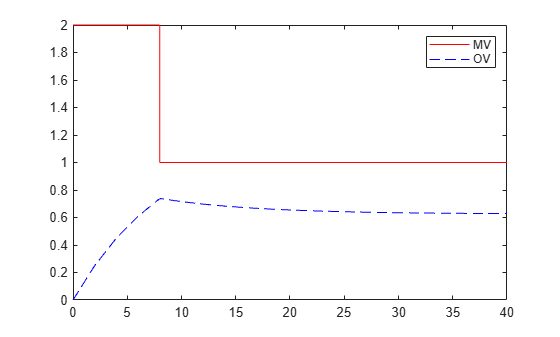# mpcmove

Compute optimal control action and update controller states

## Syntax

``mv = mpcmove(MPCobj,xc,ym,r,v)``
``[mv,info] = mpcmove(MPCobj,xc,ym,r,v)``
``[___] = mpcmove(___,options)``

## Description

Use this command to simulate an MPC controller in closed-loop with a discrete-time plant model. Call `mpcmove` repeatedly in a for loop to calculate the manipulated variable and update the controller states at each time step.

example

````mv = mpcmove(MPCobj,xc,ym,r,v)` returns the optimal move `mv` and updates the states xc of the controller `MPCobj`.The manipulated variable `mv` at the current time is calculated given: the controller object, `MPCobj`,the current estimated extended state, `xc`,the measured plant outputs, `ym`,the output references, `r`,and the measured disturbance input, `v`. The updated controller state is returned in the input argument `xc`.```

example

````[mv,info] = mpcmove(MPCobj,xc,ym,r,v)` returns additional information about the optimization problem solved to calculate `mv`.```
````[___] = mpcmove(___,options)` overrides default constraints and weights in `MPCobj` with the values specified in `Options`, an `mpcmoveopt` object. Use `Options` to provide run-time adjustment of constraints and weights during the closed-loop simulation.```

## Examples

collapse all

Perform closed-loop simulation of a plant with one MV and one measured OV.

Define a plant model and create a model predictive controller with MV constraints.

```ts = 2; Plant = ss(0.8,0.5,0.25,0,ts); MPCobj = mpc(Plant);```
```-->The "PredictionHorizon" property of "mpc" object is empty. Trying PredictionHorizon = 10. -->The "ControlHorizon" property of the "mpc" object is empty. Assuming 2. -->The "Weights.ManipulatedVariables" property of "mpc" object is empty. Assuming default 0.00000. -->The "Weights.ManipulatedVariablesRate" property of "mpc" object is empty. Assuming default 0.10000. -->The "Weights.OutputVariables" property of "mpc" object is empty. Assuming default 1.00000. ```
```MPCobj.MV(1).Min = -2; MPCobj.MV(1).Max = 2;```

Initialize, and return an handle object to the controller state, for simulation. Use the default state properties.

`xc = mpcstate(MPCobj);`
```-->Assuming output disturbance added to measured output channel #1 is integrated white noise. -->The "Model.Noise" property of the "mpc" object is empty. Assuming white noise on each measured output channel. ```

Set the reference signal. There is no measured disturbance.

`r = 1;`

Simulate the closed-loop response by calling `mpcmove` iteratively.

```t = [0:ts:40]; N = length(t); y = zeros(N,1); u = zeros(N,1); for i = 1:N % simulated plant and predictive model are identical y(i) = 0.25*xc.Plant; u(i) = mpcmove(MPCobj,xc,y(i),r); end```

`y` and `u` store the OV and MV values, respectively.

Analyze the result.

```[ts,us] = stairs(t,u); plot(ts,us,'r-',t,y,'b--') legend('MV','OV')```Modify the MV upper bound as the simulation proceeds using an `mpcmoveopt` object.

```MPCopt = mpcmoveopt; MPCopt.MVMin = -2; MPCopt.MVMax = 2;```

Simulate the closed-loop response and introduce the real-time upper limit change at eight seconds (the fifth iteration step).

```xc = mpcstate(MPCobj); y = zeros(N,1); u = zeros(N,1); for i = 1:N % simulated plant and predictive model are identical y(i) = 0.25*xc.Plant; if i == 5 MPCopt.MVMax = 1; end u(i) = mpcmove(MPCobj,xc,y(i),r,[],MPCopt); end```

Analyze the result.

```[ts,us] = stairs(t,u); plot(ts,us,'r-',t,y,'b--') legend('MV','OV')```Define a plant model.

```ts = 2; Plant = ss(0.8,0.5,0.25,0,ts);```

Create a model predictive controller with constraints on both the manipulated variable and the rate of change of the manipulated variable. The prediction horizon is `10` intervals, and the control horizon is blocked.

`MPCobj = mpc(Plant,ts,10,[2 3 5]);`
```-->The "Weights.ManipulatedVariables" property of "mpc" object is empty. Assuming default 0.00000. -->The "Weights.ManipulatedVariablesRate" property of "mpc" object is empty. Assuming default 0.10000. -->The "Weights.OutputVariables" property of "mpc" object is empty. Assuming default 1.00000. ```
```MPCobj.MV(1).Min = -2; MPCobj.MV(1).Max = 2; MPCobj.MV(1).RateMin = -1; MPCobj.MV(1).RateMax = 1;```

Initialize (and return an handle to) the controller internal state for simulation.

`xc = mpcstate(MPCobj);`
```-->Assuming output disturbance added to measured output channel #1 is integrated white noise. -->The "Model.Noise" property of the "mpc" object is empty. Assuming white noise on each measured output channel. ```
```xc.Plant = 2.8; xc.LastMove = 0.85;```

Compute the optimal control move at the current time.

```y = 0.25*xc.Plant; r = 1; [u,Info] = mpcmove(MPCobj,xc,y,r);```

Analyze the predicted optimal sequences.

```[ts,us] = stairs(Info.Topt,Info.Uopt); plot(ts,us,'r-',Info.Topt,Info.Yopt,'b--') legend('MV','OV')````plot` ignores `Info.Uopt(end)` as it is `NaN`.

Examine the optimal cost.

`Info.Cost`
```ans = 0.0793 ```

## Input Arguments

collapse all

Model predictive controller, specified as an MPC controller object. To create an MPC controller, use `mpc`.

Current controller state handle, specified as an `mpcstate` object.

Before you begin a simulation with `mpcmove`, initialize the controller, and return an handle to its state using ```xc = mpcstate(MPCobj)```. Then, modify the default properties of `xc` as appropriate. `mpcmove` modifies the controller state. The handle object `xc` always reflect the current (updated) state of the controller.

If you are using default state estimation, `mpcmove` expects `xc` to represent `xc[n|n-1]`. The `mpcmove` command updates the state values in the previous control interval with that information. Therefore, you should not programmatically update `xc` at all. The default state estimator employs a steady-state Kalman filter.

If you are using custom state estimation, `mpcmove` expects `xc` to represent `xc[n|n]`. Therefore, prior to each `mpcmove` command, you must set `xc.Plant`, `xc.Disturbance`, and `xc.Noise` to the best estimates of these states (using the latest measurements) at the current control interval.

Current measured output values at time k, specified as a column vector of length Nym, where Nym is the number of measured outputs.

If you are using custom state estimation, set `ym = []`.

Plant output reference values, specified as a p-by-Ny array, where p is the prediction horizon of `MPCobj` and Ny is the number of outputs. Row `r(i,:)` defines the reference values at step i of the prediction horizon.

`r` must contain at least one row. If `r` contains fewer than p rows, `mpcmove` duplicates the last row to fill the p-by-Ny array. If you supply exactly one row, therefore, a constant reference applies for the entire prediction horizon.

To implement reference previewing, which can improve tracking when a reference varies in a predictable manner, `r` must contain the anticipated variations, ideally for p steps.

Current and anticipated measured disturbances, specified as a (p+1)-by-Nmd array, where p is the prediction horizon of `MPCobj` and Nmd is the number of measured disturbances. The first row of `v` specifies the current measured disturbance values. Row `v(i+1,:)` defines the anticipated disturbance values at step i of the prediction horizon.

Modeling of measured disturbances provides feedforward control action. If your plant model does not include measured disturbances, use `v` = `[]`.

If your model includes measured disturbances, `v` must contain at least one row. If `v` contains fewer than p+1 rows, `mpcmove` duplicates the last row to fill the (p+1)-by-Nmd array. If you supply exactly one row, a constant measured disturbance applies for the entire prediction horizon.

To implement disturbance previewing, which can improve tracking when a disturbance varies in a predictable manner, `v` must contain the anticipated variations, ideally for p steps.

Run-time options, specified as an `mpcmoveopt` object. Use `options` to override selected properties of `MPCobj` during simulation. These options apply to the current `mpcmove` time instant only. Using `options` yields the same result as redefining or modifying `MPCobj` before each call to `mpcmove`, but involves considerably less overhead. Using `options` is equivalent to using an MPC Controller Simulink® block in combination with optional input signals that modify controller settings, such as MV and OV constraints.

## Output Arguments

collapse all

Optimal manipulated variable moves, returned as a column vector of length Nmv, where Nmv is the number of manipulated variables.

If the controller detects an infeasible optimization problem or encounters numerical difficulties in solving an ill-conditioned optimization problem, `mv` remains at its most recent successful solution, `xc.LastMove`.

Otherwise, if the optimization problem is feasible and the solver reaches the specified maximum number of iterations without finding an optimal solution, `mv`:

• Remains at its most recent successful solution if the `Optimizer.UseSuboptimalSolution` property of the controller is `false`.

• Is the suboptimal solution reached after the final iteration if the `Optimizer.UseSuboptimalSolution` property of the controller is `true`. For more information, see Suboptimal QP Solution.

Solution details, returned as a structure with the following fields.

Predicted optimal manipulated variable adjustments (moves), returned as a (p+1)-by-Nmv array, where p is the prediction horizon and Nmv is the number of manipulated variables.

`Uopt(i,:)` contains the calculated optimal values at time `k+i-1`, for `i = 1,...,p`, where `k` is the current time. The first row of `Info.Uopt` contains the same manipulated variable values as output argument `mv`. Since the controller does not calculate optimal control moves at time `k+p`, `Uopt(p+1,:)` is equal to `Uopt(p,:)`.

Optimal output variable sequence, returned as a (p+1)-by-Ny array, where p is the prediction horizon and Ny is the number of outputs.

The first row of `Info.Yopt` contains the calculated outputs at time `k` based on the estimated states and measured disturbances; it is not the measured output at time `k`. `Yopt(i,:)` contains the predicted output values at time `k+i-1`, for ```i = 1,...,p+1```.

`Yopt(i,:)` contains the calculated output values at time `k+i-1`, for `i = 2,...,p+1`, where `k` is the current time. `Yopt(1,:)` is computed based on the estimated states and measured disturbances.

Optimal prediction model state sequence, returned as a (p+1)-by-Nx array, where p is the prediction horizon and Nx is the number of states in the plant and unmeasured disturbance models (states from noise models are not included).

`Xopt(i,:)` contains the calculated state values at time `k+i-1`, for `i = 2,...,p+1`, where `k` is the current time. `Xopt(1,:)` is the same as the current states state values.

Time intervals, returned as a column vector of length p+1. `Topt(1)` = 0, representing the current time. Subsequent time steps `Topt(i)` are given by `Ts*(i-1)`, where `Ts = MPCobj.Ts` is the controller sample time.

Use `Topt` when plotting the `Uopt`, `Xopt`, or `Yopt` sequences.

Slack variable, ε, used in constraint softening, returned as `0` or a positive scalar value.

• ε = 0 — All constraints were satisfied for the entire prediction horizon.

• ε > 0 — At least one soft constraint is violated. When more than one constraint is violated, ε represents the worst-case soft constraint violation (scaled by your ECR values for each constraint).

Number of solver iterations, returned as one of the following:

• Positive integer — Number of iterations needed to solve the optimization problem that determines the optimal sequences.

• `0` — Optimization problem could not be solved in the specified maximum number of iterations.

• `–1` — Optimization problem was infeasible. An optimization problem is infeasible if no solution can satisfy all the hard constraints.

• `–2` — Numerical error occurred when solving the optimization problem.

Optimization solution status, returned as one of the following:

• `'feasible'` — Optimal solution was obtained (`Iterations` > 0)

• `'infeasible'` — Solver detected a problem with no feasible solution (`Iterations` = –1) or a numerical error occurred (`Iterations` = –2)

• `'unreliable'` — Solver failed to converge (`Iterations` = 0). In this case, if `MPCobj.Optimizer.UseSuboptimalSolution` is `false`, `u` freezes at the most recent successful solution. Otherwise, it uses the suboptimal solution found during the last solver iteration.

Objective function cost, returned as a nonnegative scalar value. The cost quantifies the degree to which the controller has achieved its objectives. For more information, see Optimization Problem.

The cost value is only meaningful when ```QPCode = 'feasible'```, or when `QPCode = 'feasible'` and `MPCobj.Optimizer.UseSuboptimalSolution` is `true`.

## Tips

• `mpcmove` updates `xc`, even though it is an input argument.

• If `ym`, `r` or `v` is specified as `[]`, `mpcmove` uses the appropriate `MPCobj.Model.Nominal` value instead.

• To view the predicted optimal behavior for the entire prediction horizon, plot the appropriate sequences provided in `Info`.

• To determine the optimization status, check `Info.Iterations` and `Info.QPCode`.

## Alternatives

Introduced before R2006a